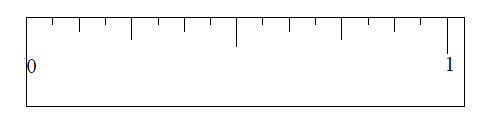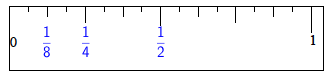### Home > MC1 > Chapter 3 > Lesson 3.2.3 > Problem3-56

3-56.

Hannah's Hardware Haven is expecting a new shipment of screws, and Hannah needs to organize a place for them in the shop. She wants to label the shelves so that the boxes of screws are arranged from shortest to longest. The shipment will include screws of the following lengths: $\frac { 3 } { 8 }$inch, $\frac { 1 } { 4 }$inch, $\frac { 7 } { 8 }$inch, $\frac { 3 } { 4 }$inch, and $\frac { 1 } { 2 }$inch. Use the enlarged ruler below to help Hannah order the labels on the shelves from least to greatest.First, it may be helpful to label the ruler with measurements you do know.

Can you find the $\frac{1}{2}$inch placement?

The ruler is now labeled with the basic measurement $\frac{1}{8},\ \frac{1}{4},\text{ and }\frac{1}{2}$.

Can you label the rest of the ruler, and then put Hannah's screws in order from least to greatest?The screws should be organized from $\frac{1}{4},\ \frac{3}{8},\ \frac{1}{2},\ \frac{3}{4}\text{ to }\frac{7}{8}$inches.